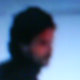möbiusIn math there is a geometric-topologic construction which is a source of fun and surprises. It is surface. It is a two dimensional object which can be embed in the three dimensional euclidean space,$\mathbb{R}^3$. It is a model to illustrate the concept of twisted bundle. In fact an$I$-bundle, an interval bundle over the circle.  The möbius band or möbius strip is the principal element to construct all the non orientable surfaces:$N_1$ the projective plane;$N_2$ the Klein bottle;$N_3$;$N_4$,… etc.

What happens if we remove the core central simple closed curve? look at the 2nd image.

I had spended many eons to popularize the symbol$M\ddot{o}$

for her. Isn’t this is beautiful?The möbius band is employed to manufature many exotic non orientable three dimensional spaces. By the record, talking about 3d spaces:$\mathbb{R}^3$ isn’t the only one.

For example$M\ddot{o}\times I$ is a solid Klein bottle and an innocent quiz is: can you see what is the bounding surface of this$I$-bundle?

The images were rendered in Mathematica v.5

La siguiente figura te explica como ver una construcción tridimensional que involucra a la banda…

Aquí estamos usando$N_2$ para la superficie de género dos no orientable,,

Filed under cucei math, fiber bundle, low dimensional topology, topology

9 responses to “möbius”

1.David D

le dejo esta animacion q se cruzo por mi camino, es del jale que me platico el martes de la banda de mobius sobre el producto cartesiano de 2 de ellas:•juanmarqz

muy bien!.. pero la botella de Klein no es el producto cartesiano de dos bandas de Möbius… más bien es la unión de dos de ellas… la unión es identificando sus respectivas fronteras… saludos

•David D

ha cierto cierto, me apen…..je, eso quice decir

2.Octavian

Is the link you give broken, or something error has happened with the wp.me service? The link you give above ends up with the page which is not found.

•qit

Andy, give me a couple of hours…

•qit
•Octavian

Ow, thank you very much, qit..

3.Octavian

Good symbol. I was confusing the first time I saw it when you wrote on my blog.

Wait, what do you mean by ‘bounding surface”? What I see is just the cylinder-like object but with a “strange twist” on one spot corresponding to the twsting of the Mobius strip.

•juanmarqz

check: http://wp.me/aAes4-jz

there, it is a raw handkrafted image of$M\ddot{o}\times I$ and it is very little difficult to see its boundary:$\partial(M\ddot{o}\times I)=M\ddot{o}\times \{0\}\cup(\partial M\ddot{o})\times I\cup M\ddot{o}\times \{1\}$ which is a Klein bottle, right?
This 3d$\partial$-manifold is a building block to assemble more complex 3-manifolds. Others are:$M\ddot{o}\stackrel{\sim}\times I,\ T\times I,\ T\stackrel{\sim}\times I$